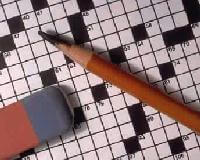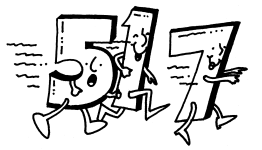Worksheets and No Prep Teaching Resources Make Puzzles Math UnitsNumber Puzzles

Runaway Math Puzzles
 Runaway Math PuzzlesKakuro Puzzles

Number Basics
Read the Number Word (up to millions)
Write the Place Value (up to Thousands)
Write the Place Value (up to millions)
Tens and Ones
Hundreds, Tens, and Ones

Counting Money Math Number Puzzles
Counting Money (Number Puzzle)
Money Word Calculations (Number Puzzle)
Combined Counting and Money Word Calculations (Number Puzzle)

Rounding
Round Whole Numbers to nearest tens, hundreds, or thousands.
Small to Very Large Numbers (Round to Largest Place Value)
Round Decimals to the Nearest Whole Number
Rounding Decimals (Mixed)
Round Decimal to the Underlined Place Value

Number Theory
Greatest Common Factor and Least Common Multiple

Numbers 0 to 6 (Top Down)
Numbers 0 to 6 (Across)
Easy Column Addition (Numbers 0 to 6)
Numbers 7 to 19 (Top Down)
Numbers 7 to 19 (Across)
Numbers 0 to 13 (Top Down)
Numbers 0 to 13 (Across)
Column Addition (Numbers 1 to 5)
Numbers 0 to 13 (Top Down)
Numbers 0 to 13 (Across)
Column Addition (Numbers 1 to 9)
Column Addition (Numbers 1 to 19)
Numbers 0 to 29 (Across)
Column Addition (4 Numbers 0 to 9)
Add 4 Numbers (Numbers 0 to 9)
Add 5 Numbers (Numbers 0 to 9)
Addition (Across, Column, and 4+ Numbers)
Addition (2 - 5 Digit Numbers)

Subtraction
Numbers 0 to 6 (Top Down)
Numbers 0 to 6 (Across)
Numbers 7 to 19 (Top Down)
Numbers 7 to 19 (Across)
Numbers 0 to 13 (Top Down)
Numbers 0 to 13 (Across)
Numbers 0 to 20 (Top Down)
Numbers 0 to 20 (Across)
Numbers 0 to 29 (Across)
2 Digit Subtraction (No Borrowing)
2 Digit Subtraction (Borrowing)
3 Digit Subtraction (No Borrowing)
3 Digit Subtraction (Borrowing)
4 Digit Subtraction
Subtraction
Subtraction (Across and Top Down)
Subtraction (2 - 5 Digit Numbers)
Subtraction (Large Numbers)

Multiplication
Multiplication Basics (Number 2)
Multiplication Basics (Number 3)
Multiplication Basics (Number 4)
Multiplication Basics (Number 5)
Multiplication Basics (Number 6)
Multiplication Basics (Number 7)
Multiplication Basics (Number 8)
Multiplication Basics (Number 9)
Multiplication Basics (Number 10)
Multiplication Basics (Number 11)
Multiplication Basics (Number 12)
Multiplication Basics (Number 13)
Multiplication Basics (Number 14)
Multiplication (Mixed/Difficult)
Multiplication (Across, Numbers 2-12)
Multiplication (Numbers 2-12)
Multiplication (Fill in the Missing Factor)
Multiplication (2 Digits by Numbers 2-9)
Multiplication (3 Digits by Numbers 2-9)
Multiplication (2 Digits by 2 Digits)
Multiplication (Mixed)
Multiplication (2-3 Digits by a 1 Digit Number)
Multiplication (3 Digits by 2 Digits)
Multiplication (4 Digits by 2 Digits)
Multiplication (4 by 1, 4 by 2, and 5 by 2 Digits)
Multiplication (3-6 Digits by 2 Digits)
Multiplication (bigger numbers)

Division
Introduction to Division (graphics)
How many 2's are there in 26...
Division (Across Divide by 2 - 12)
Division (Fill in the Missing Factor)
Division (Small Numbers - No Remainders)
Division (Bigger Numbers - No Remainders)
Division (Remainders)
Division (Remainders 3 Digits by 2-12)
Division (No Remainders 3-4 Digits by 2-12)
Division (Remainders 3-4 Digits by 2-12)
Division (mixed remainders and no remainders)

Addition and Subtraction (Numbers 0 to 6)
Addition and Subtraction (Numbers 0 to 13)
Addition and Subtraction (Numbers 7 to 19)
Addition and Subtraction (Numbers 0 to 20)
Addition and Subtraction - All Across (Numbers 0 to 20)
Addition and Subtraction - All Across (Numbers 0 to 29)
Grouped Multiplication (includes -,+ and 3+ numbers)
Grouped Division (includes -,+ and 3+ numbers)

Decimals
Round to the Nearest Whole Number
Rounding Decimals (Mixed)
Round Decimal to the Underlined Place Value
Multiplication and Division (no remainders)
Multiplication and Division (more difficult - no remainders)
Multiplication and Division (with remainders)

Addition, Subtraction, Multiplication, Division Math Money CrossNumber Puzzles!
Money Subtraction (Number Puzzle)
Money Addition and Subtraction (Number Puzzle)
Money Multiplication (Number Puzzle)
Money Division (Number Puzzle)
Money Multiplication and Division (Number Puzzle)
Money Addition, Subtraction, Multiplication and Division (Number Puzzle)

Ratios
Introduction to Ratios
Solving Proportions
Write each decimal as a percent
Write each fraction as a decimal
Fractional Parts
Percent of a number

Measurement
English Length
English Weight
Time Conversions
Mixed English Conversions
Metric Length
Metric Weight
Metric Capacity
Mixed Metric Conversions
Temperature Conversions
Mixed Metric Conversions with Decimals

Measurement Arithmetic
Multiplication of English Measurements
Mixed English Measurement Arithmetic

Fractions
Writing Tenths Fractions and Decimals
Writing Hundredths Fractions and Decimals
Reduce Improper Fractions to Lowest Terms
Reduce to Lowest Terms
Write Fraction as a Decimal (10 and 100 Denominators)
Write Fraction as a Decimal
Write Mixed Number (includes Number Words) as a Decimal
Write Decimal as a Fraction
Fill in the Missing Numerator or Denominator
Subtraction (Like Denominators)
Addition (Like Denominators - Reduce to Lowest Terms)
Subtraction (Like Denominators- Reduce to Lowest Terms)
Subtraction (Different Denominators)
Multiplication
Multiplication (Mixed Fractions)
Division
Division (Mixed Fractions)
Multiplication and Division

Order of Operations
Order of Operations (3 Numbers Only)
Order of Operations (No Exponents)
Order of Operations (Some Exponents)

Integers
Introduction to Integers
Write the opposite of each integer
Basic Absolute Number Worksheet
Subtraction
Multiplication
Division
Multiplication and Division
Mixed
Introduction to Absolute Values
Multiplication and Division (more...)

Algebra
Translating Verbal Statements into Equations Crossword Puzzle
Solving Simple Equations Using Inverse Operations Crossword Puzzle
Solving Addition and Subtraction Equations Crossword Puzzle
Solving Multiplication and Division Equations Crossword Puzzle
Solving Equations with 2 to 4 Numbers Crossword Puzzle
More 2 to 4 Number Equations Crossword Puzzle
Equations Final Review Crossword Puzzle!

Exponent Worksheets Crossword Puzzles
Evaluate Exponents Crossword Puzzle
Multiplying Powers Crossword Puzzle
Multiplying Powers with Unknowns (Monomials) Crossword Puzzle
Dividing Powers Crossword Puzzle
Dividing Powers with Unknowns (Monomials) Crossword Puzzle
Multiplying Monomials (No Negatives) Crossword Puzzle
Multiplying Monomials (Negatives) Crossword Puzzle
Multiplying Monomials (Negatives and Power-Of-A-Power or Power-Of-A-Product) Crossword Puzzle
Division of Monomials Crossword Puzzle
Division of Monomials (Includes Multiplication) Crossword Puzzle
Division of Monomials (Multiplication and Power-Of-A-Power or Power-Of-A-Product) Crossword Puzzle
Scientific Notation: Rewrite in Scientific Notation Crossword Puzzle
Scientific Notation: Rewrite in Decimal Notation Crossword Puzzle
Scientific Notation: Multiplication Crossword Puzzle
Scientific Notation: Division Crossword Puzzle
Exponents Final Review Crossword Puzzle!

Systems of Linear Equations and Inequalities Crossword Puzzles
Linear Systems - Solve by using the substitution method (in Ax + By = C format) Crossword Puzzle
Linear Systems - Solve by using the substitution method Crossword Puzzle
Linear Systems - Solve by using the elimination method (in Ax + By = C format) Crossword Puzzle
Linear Systems - Solve by using the elimination method Crossword Puzzle
Linear Systems - Solve by using any method (in Ax + By = C format) Crossword Puzzle
Linear Systems - Solve by using any method Crossword Puzzle
Inequalities - Solve each system of inequalities by graphing (3 vertices) Crossword Puzzle
Inequalities - Solve each system of inequalities by graphing (4 vertices) Crossword Puzzle
Inequalities - Solve each system of inequalities by graphing (3-4 vertices) Crossword Puzzle
Linear Programming - Find the minimum and maximum (3 vertices) Crossword Puzzle
Linear Programming - Find the minimum and maximum (4 vertices) Crossword Puzzle
Linear Programming - Find the minimum and maximum (3-4 vertices) Crossword Puzzle
Systems of Linear Equations in Three Variables - Solve (easier problems) Crossword Puzzle
Systems of Linear Equations in Three Variables - Solve Crossword Puzzle
Review Crossword Puzzle

Polynomial Crossword Puzzles
Subtracting Polynomials Crossword Puzzle
Multiplication (Monomial by Polynomial) Crossword Puzzle
Multiplication using FOIL (Binomial by Binomial) Crossword Puzzle
Multiplication (Polynomial by Polynomial) Crossword Puzzle
Dividing a Monomial by a Monomial Crossword Puzzle
Dividing a Polynomial by a Monomial Crossword Puzzle
Mixing Addition, Multiplication, and Division in one problem Crossword Puzzle
A mix of all the different operation worksheets Crossword Puzzle
Common Factors: Factor out the GCF Crossword Puzzle
Common Factors: Write as a Product of Two Binomials Crossword Puzzle
Factoring: Perfect-Square Trinomials Crossword Puzzle
Factoring: Perfect-Squares (more difficult) Crossword Puzzle